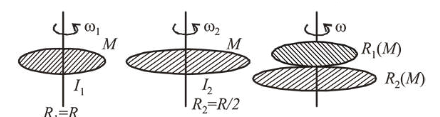# A circular disc of mass M and radius R is rotating about

Question:

A circular disc of mass $M$ and radius $R$ is rotating about its axis with angular speed $\omega_{1}$. If another stationary disc

having radius $\frac{R}{2}$ and same mass $M$ is dropped co-axially

on to the rotating disc. Gradually both discs attain constant angular speed $\omega_{2}$. The energy lost in the process is $p \%$ of the initial energy. Value of $p$ is__________

Solution:

(20)

As we know moment of inertia disc, $I_{\mathrm{disc}}=\frac{1}{2} M R^{2}$Using angular momentum conservation

$I_{1} \omega_{1}+I_{2} \omega_{2}=\left(I_{1}+I_{2}\right) \times \omega_{f}$

$\frac{M R^{2}}{2} \times \omega+0=\left(\frac{M R^{2}}{2}+\frac{M R^{2}}{8}\right) \omega_{f} \Rightarrow \omega_{f}=\frac{4}{5} \omega$

Initial K.E., $K_{i}=\frac{1}{2} I \omega^{2}=\frac{1}{2}\left(\frac{M R^{2}}{2}\right) \omega^{2}=\frac{M R^{2} \omega^{2}}{4}$

Final K.E., $K_{f}=\frac{1}{2}\left(\frac{M R^{2}}{2}+\frac{M R^{2}}{8}\right) \frac{16}{25} \omega^{2}=\frac{M R^{2} \omega^{2}}{5}$

Percentage loss in kinetic energy $\%$ loss

$=\frac{\frac{M R^{2} \omega^{2}}{4}-\frac{M R^{2} \omega^{2}}{5}}{\frac{M R^{2} \omega^{2}}{4}} \times 100=20 \%=P \%$

Hence, value of $P=20$